The ARIMA Procedure
 Forecasting Details

If the model has input variables, a forecast beyond the end of the data for the input variables is possible only if univariate ARIMA models have previously been fit to the input variables or future values for the input variables are included in the DATA= data set.

If input variables are used, the forecast standard errors and confidence limits of the response depend on the estimated forecast error variance of the predicted inputs. If several input series are used, the forecast errors for the inputs should be independent; otherwise, the standard errors and confidence limits for the response series will not be accurate. If future values for the input variables are included in the DATA= data set, the standard errors of the forecasts will be underestimated since these values are assumed to be known with certainty.

The forecasts are generated using forecasting equations consistent with the method used to estimate the model parameters. Thus, the estimation method specified in the ESTIMATE statement also controls the way forecasts are produced by the FORECAST statement. If METHOD=CLS is used, the forecasts are infinite memory forecasts, also called conditional forecasts. If METHOD=ULS or METHOD=ML, the forecasts are finite memory forecasts, also called unconditional forecasts. A complete description of the steps to produce the series forecasts and their standard errors by using either of these methods is quite involved, and only a brief explanation of the algorithm is given in the next two sections. Additional details about the finite and infinite memory forecasts can be found in Brockwell and Davis (1991). The prediction of stationary ARMA processes is explained in Chapter 5, and the prediction of nonstationary ARMA processes is given in Chapter 9 of Brockwell and Davis (1991).

Infinite Memory Forecasts

If METHOD=CLS is used, the forecasts are infinite memory forecasts, also called conditional forecasts. The term conditional is used because the forecasts are computed by assuming that the unknown values of the response series before the start of the data are equal to the mean of the series. Thus, the forecasts are conditional on this assumption.

The series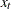can be represented as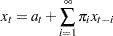where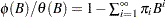.

The k -step forecast of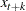is computed as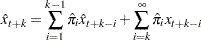where unobserved past values ofare set to zero and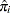is obtained from the estimated parameters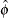and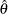.

Finite Memory Forecasts

For METHOD=ULS or METHOD=ML, the forecasts are finite memory forecasts, also called unconditional forecasts. For finite memory forecasts, the covariance function of the ARMA model is used to derive the best linear prediction equation.

That is, the k-step forecast of, given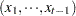, is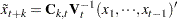where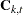is the covariance ofand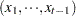and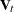is the covariance matrix of the vector.andare derived from the estimated parameters.

Finite memory forecasts minimize the mean squared error of prediction if the parameters of the ARMA model are known exactly. (In most cases, the parameters of the ARMA model are estimated, so the predictors are not true best linear forecasts.)

If the response series is differenced, the final forecast is produced by summing the forecast of the differenced series. This summation and the forecast are conditional on the initial values of the series. Thus, when the response series is differenced, the final forecasts are not true finite memory forecasts because they are derived by assuming that the differenced series begins in a steady-state condition. Thus, they fall somewhere between finite memory and infinite memory forecasts. In practice, there is seldom any practical difference between these forecasts and true finite memory forecasts.Previous Page | Next Page | Top of Page